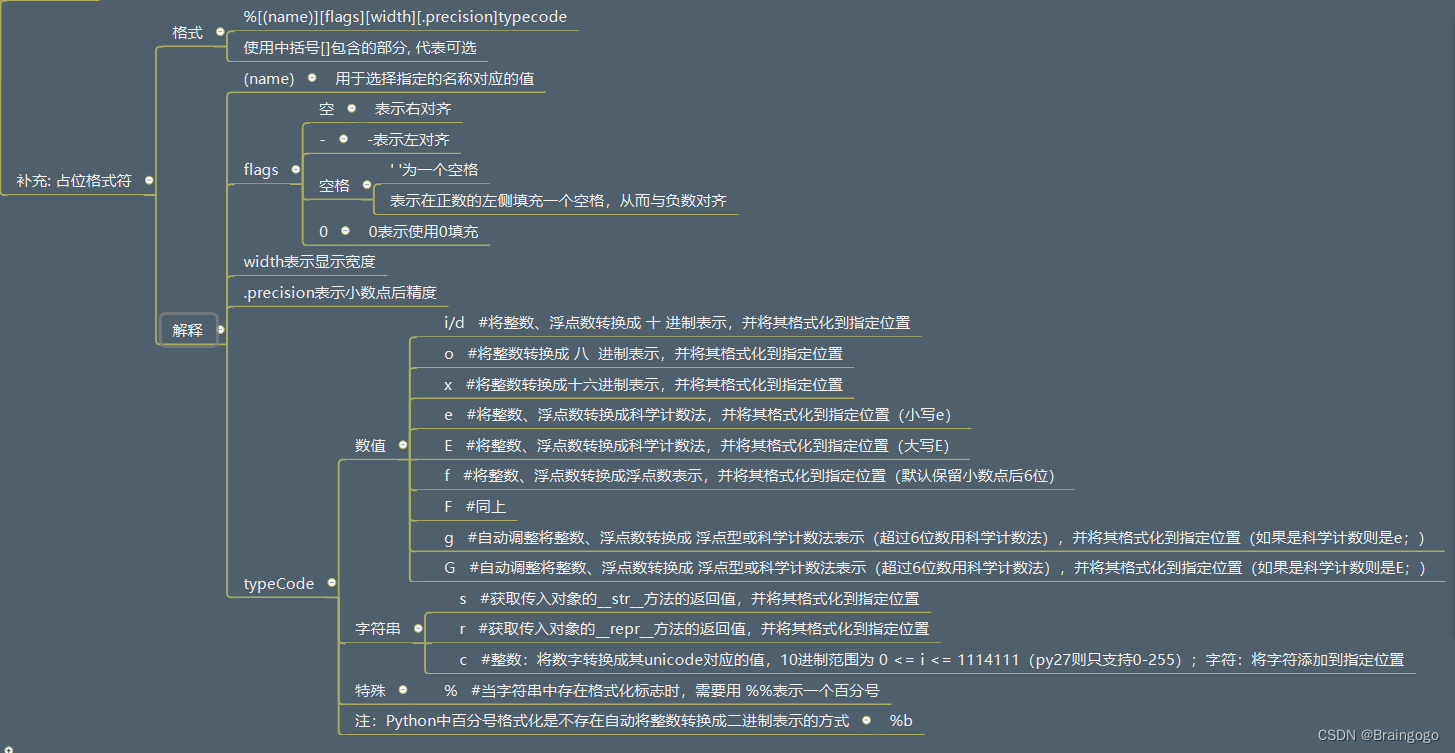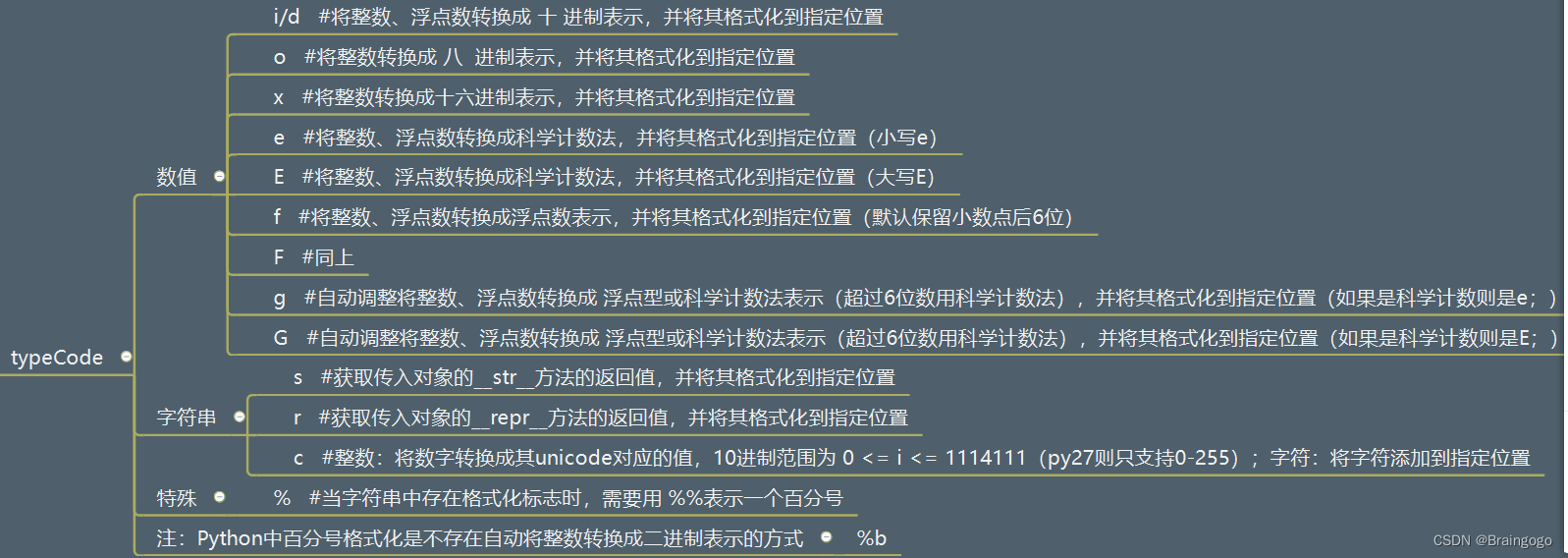### Python的占位格式符对于print函数里的语句

`` print("我的名字是%s, 年龄是%d"%(name, age))``

``%[(name)][flags][width][.precision]typecode``

[(name)]:

``(name) 表示, 根据, 制定的名称(key), 查找对应的值, 格式化到字符串当中mathScore = 59englishScore = 58 print("我的数学分数是%d, 英文的分数是: %d"%(mathScore, englishScore)) print("我的数学分数是%d, 英文的分数是: %d"%(englishScore, mathScore)) print("我的数学分数是%(ms)d, 英文的分数是: %(es)d"%({"es": englishScore, "ms": mathScore}))``

[width]：

``width , 表示, 占用的宽度 print("%10d" % mathScore)``

[flags]:

`` min = 5 sec = 18# # 05:08 print("%02d:%02d"%(min, sec))``

[.precision]:

typecode：``score=65print("%d%"%score)``

``​score=65print("%d%%"%score) ​``